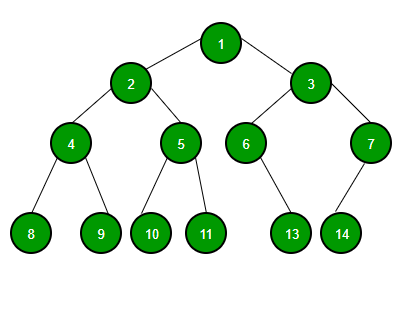# Binary Tree Data Structure

• Last Updated : 08 May, 2023

## What is Binary Tree Data Structure?

Binary Tree is defined as a tree data structure where each node has at most 2 children. Since each element in a binary tree can have only 2 children, we typically name them the left and right child.## Binary Tree Representation

A Binary tree is represented by a pointer to the topmost node (commonly known as the “root”) of the tree. If the tree is empty, then the value of the root is NULL. Each node of a Binary Tree contains the following parts:

1. Data
2. Pointer to left child
3. Pointer to right child

## Basic Operation On Binary Tree:

• Inserting an element.
• Removing an element.
• Searching for an element.
• Traversing the tree.

## Auxiliary Operation On Binary Tree:

• Finding the height of the tree
• Find the level of a node of the tree
• Finding the size of the entire tree.

Topic :

Introduction :

Basic Operations on Binary Tree:

Some other important Binary Tree Traversals :

Must solve Standard Problems on Binary Tree Data Structure: